## Constant Current Source in Differential Amplifier:

An ideal current source provides a constant current regardless of the load connected to it. There are many applications in electronics for a circuit that provides a constant current with a very high impedance. Constant Current Source in Differential Amplifier can be built using FET devices, bipolar devices, and a combination of these components. There are circuits used in discrete form and others more suitable for operation in ICs. Some forms of both types will be considered here.

### JFET Constant Current Source:

Figure 20.16(a) depicts a simple JFET current source. With VGS set to zero volt, the drain current ID is fixed at IDSS = 10 mA. The device operates like a current source of magnitude 10 mA.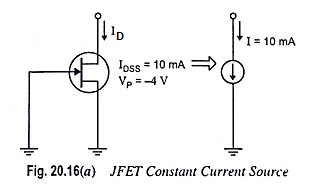### BJT Constant Current Source:

There are different methods of connecting BJTs in circuit that acts as a constant current source. Figure 20.16(b) depicts a circuit using an NPN transistor and a few resistors for operation as a constant current source in differential amplifier.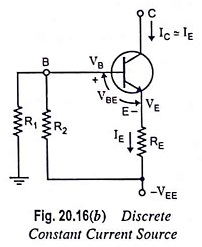Constant current lC is given by the following equation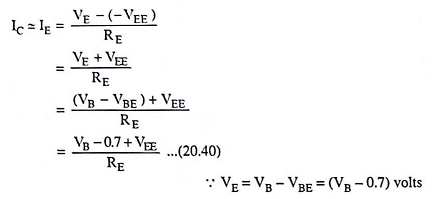where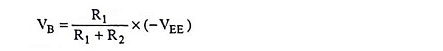assuming the base input impedance much larger than R1 or R2.

### Transistor/Zener Constant Current Source:

If resistor R2 is replaced with a zener diode in the circuit depicted in Fig. 20.16(b), an improved constant current source is obtained in Fig. 20.17.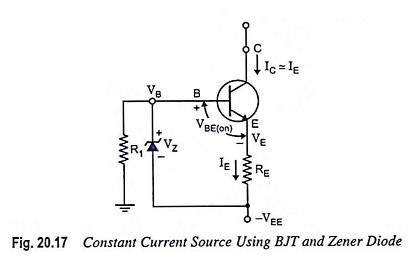The zener diode results in a constant current I given by the equation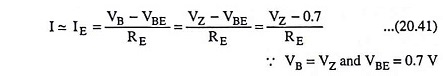The noteworthy point is that the constant current delivered by the circuit shown in Fig. 20.18 depends on the zener diode voltage, which remains quite constant and the emitter resistor RE. The voltage supply VEE has no effect on the magnitude of current I delivered by the circuit.

Scroll to Top